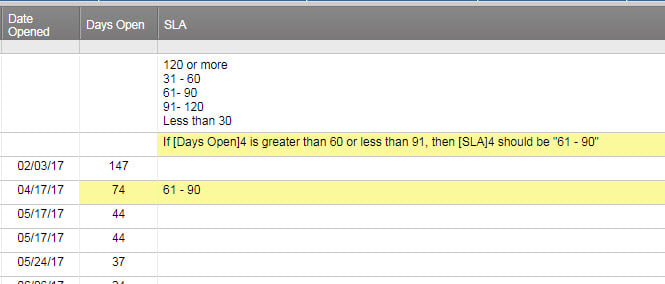# Seeking correct formula for "If greater than, but less than" statement.

If cell A is > 30, but < 61, then cell B is "31 - 60"

Tags:

• I finally figured it out: =IF(AND([Days Open]4 > 60, [Days Open]4 < 91), "61-90")

• Try this... You only need brackets around the column names if they have spaces. the 1 after the column name = the row you are are specifying.

=IF(And([Column A Name]1=>30, [Column B Name]1=<61), "31-60", "")

If the item doesn't meet your criteria then the result will be blank.

• Mike, Thanks for the reply.  I explained problem incorrectly.

cell A15 has the number 31 in it.

I need cell B15 to determine if cell A15 has a number in it that is greater than 30, but less than 61.

If it does, then I want B15 to say "31-60"

• While Excell and Google Sheets reference columns with Letters, formulas in Smartsheet reference the column names. Hence A15 would be [Column A Name]15 if there are spaces in the column name or ColumnName15 if there are no spaces.

See the following examples and replace Column A name is the name of Column A.

=IF(And([Column A Name]15=>30, [Column B Name]15=<61), "31-60", "")

=IF(And(ColumnAName15=>30, ColumnBName15=<61), "31-60", "")

• I understand about the columns... it was a poor example to use.

But the formula isn't working...and I'm unclear why you placed Column B Name where you did?  In my mind, that should also be Column A Name. Column B Name is where I want the text to go based on Column A....but that didn't work either.  Both produced error: #INVALID OPERATION

IF(And([Column A Name]15=>30, [Column B Name]15=<61), "31-60", "")

Maybe the attached screenshot will help?  If not, I wont bug you anymore• I finally figured it out: =IF(AND([Days Open]4 > 60, [Days Open]4 < 91), "61-90")

• Yep - sorry I got confused by the Column A and Column B thing.Good job. Glad I helped get you onto the right track!If you want the formula to give you the run down for each of them, nest the formulas...

=IF([Days Open]4>30,"Less than 30", IF(AND([Days Open]4 > 30, [Days Open]4 < 61), "30-60", IF(AND([Days Open]4 > 60, [Days Open]4 < 91), "61-90", IF(AND([Days Open]4 > 90, [Days Open]4 < 120), "91-120", IF([Days Open]4 > 120, "Greater than 120")))))

• I need to slow down. Sheesh. Thanks for catching those errors, Craig. Good thinking.

• A little testing goes a long way, Mike. I'm guilty of sacrificing quality for speed as well, but have been burned so often in my previous line of work, I get a physical pain when I know I am doing it.

Craig

• Trying to have a column post a statement based on another columns %

<1% Not Started

100% Complete

>1% thru <100% In Progress

I can get the not started and Complete to work but I get an error for the in-between

Here is the formula I have used

=IF([% Complete]90 =0, "Not Started", IF([% Complete]90 = 1, "Complete", IF(AND([% Complete]90, >.01, [% Complete]90, <.99, "In Progress"))))

## Help Article Resources

Want to practice working with formulas directly in Smartsheet?

Check out the Formula Handbook template!